# Working with Paths

A path is a set of geometric elements (curves, text, and graphics shapes) that can be used as a single object. There are two types of paths: closed, where the starting and ending points are at the same location, and open, where these points have different coordinates.

Graphics Mill allows using paths for the following major operations: to draw/fill a path on the Graphics, to draw a text string along a path, and to use a path as the clipping path for cutting out the image outside of it (to learn more about clipping path read Clipping Path paragraph). The latter requires a closed path. If you are not familiar with such aspects as Graphics or clipping path, read the Graphics. Drawing Images and Geometric Shapes article; to learn how to draw the text using the drawing engine, read the Drawing Text article.

There are two ways to create a path: copy an existing path or draw it from scratch.

## Copying Existing Paths

If you want to create a path from an existing one, you can use the static Path.Create(GraphicsPath) method that allows initializing a path from Drawing2D.GraphicsPath or ClippingPath.Path. The snippet below demonstrates how to extract a path from ClippingPath.Path stored in Adobe Image Resource Blocks (to learn more about clipping path read the Clipping Path article). Notice that ClippingPath.Path is represented in relative coordinates, therefore you need to specify the height and width of the original:

C#
```using (var reader = new JpegReader(@"Images\1.jpg"))
{
}
```

## Creating New Paths

Creating a path means creating a number of path segments that can be defined as lines, curves, or shapes. Let us discuss how to work with each segment in more detail:

### Line Segment

A line segment is a line defined by two points. The first point is the last point that was added to the path, whereas the second point is the one passed to the LineTo(PointF) method. Thus, if the path is empty, it does not contain any segment, and you need to use the MoveTo(PointF) method to add a starting point to the path. Also do not forget to call MoveTo(PointF) in case the last path segment was a shape (rectangle, ellipse, etc.). Using the LineTo(PointF) method you can add line segments one by one. The following snippet illustrates how to draw a line segment:

C#
```var path = new Path();
path.MoveTo(10, 10);
path.LineTo(70, 60);
```

This snippet creates the line illustrated on the following graph: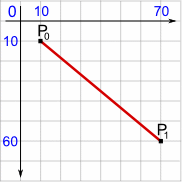### Curve Segment

The API allows you to add either a quadratic or a cubic Bezier segment to a path. Both of the curves are created using the CurveTo Method. Before adding a curve to an empty path you need to add the starting point to the path as we did it for line segments.

The quadratic Bezier segment is a curve created via the CurveTo(PointF, PointF) method. This method adds a curve starting at point P0 and arriving to P2 coming from the direction of P1. P0 is the last point added to the path, while P1 and P2 are passed to CurveTo. The following snippet demonstrates how to create the quadratic Bezier segment:

C#
```var path = new Path();
path.MoveTo(10, 60);
path.CurveTo(new System.Drawing.PointF(40, 20), new System.Drawing.PointF(70, 60));
```

This snippet creates the line illustrated on the following graph: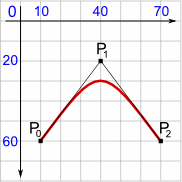Cubic Bezier

The cubic Bezier segment is a curve created via the CurveTo(PointF, PointF, PointF) method. This method adds a curve starting at the point P0, arriving at P3 coming from the direction of P1 and P2. P0 is the last point added to the path and P1, P2, and P3 are passed to CurveTo as arguments. The following snippet demonstrates how to create the cubic Bezier segment:

C#
```var path = new Path();
path.MoveTo(10, 60);
path.CurveTo(new System.Drawing.PointF(40, 20), new System.Drawing.PointF(70, 60), new System.Drawing.PointF(100, 20));
```

This snippet creates the line illustrated on the following graph: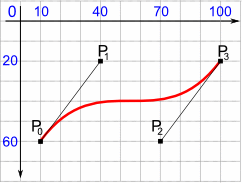### Figure Segment

A figure is a closed path segment delineating an ellipse or rectangle. To create ellipse and rectangle segments use the DrawEllipse(RectangleF) and DrawRectangle(RectangleF) methods, respectively. Unlike the Line and Curve segments, figure segments do not require adding a starting point to empty paths before creating a segment. Also, figure segments may have no links with other segments in the path. The following snippet demonstrates how to create the figure segments:

C#
```using (var bitmap = new Bitmap(160, 120, PixelFormat.Format24bppRgb, RgbColor.White))
using (var path = new Path())
{
path.DrawEllipse(new System.Drawing.Rectangle(10, 10, 70, 70));
path.DrawRectangle(new System.Drawing.RectangleF(75, 75, 75, 35));
graphics.DrawPath(new Pen(RgbColor.Green), path);
bitmap.Save(@"Images\Output\out.png");
}
```

This snippet utilizes the Graphics.DrawPath(Pen, Path) method for drawing a path on a Graphics object. After launching this snippet you will see the following result: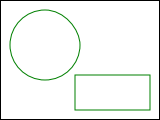You can also add a text outline to a path (for more information about text objects, see the Drawing Text article). The following snippet demonstrates how to create a path from the "Example" text:

C#
```using (var bitmap = new Bitmap(160, 120, PixelFormat.Format24bppRgb, RgbColor.White))
using (var path = new Path())
{
path.DrawText(new PlainText("Example", FontRegistry.Installed.CreateFont("Arial", 26, 96, 96), 15, 75));
graphics.DrawPath(new Pen(RgbColor.Green), path);
bitmap.Save(@"Images\Output\out.png");
}
```

As the result you will see the following path:In addition, you can create a path segment from an existing AdvancedDrawing.Path using the Path.DrawPath(Path) method:

C#
```var path = new Path();
path.DrawPath(existedPath);
```

## Operations on Path

There are a number of operations that can be performed on paths: you can transform, close, convert to GraphicsPath, or serialize paths.

### Transforming Path

The Path class provides the ApplyTransform(Matrix) method that changes a path according to a specified Matrix instance. Matrix is a transformation matrix representing a geometric transform such as rotating, scaling, translating, etc. The following snippet illustrates how to downsize the path twice as much as it originally was and then rotate it:

C#
```using (var reader = new JpegReader(@"Images\1.jpg"))
{
var m = new System.Drawing.Drawing2D.Matrix();
m.Scale(0.5f, 0.5f);
m.Rotate(30);
path.ApplyTransform(m);
}
```

### Closing Path

Earlier we discussed that a path can be open or closed. You can use the Path.Close() method to close a path. This method copies the starting point to the ending one. The following snippet demonstrates how to close the path:

C#
```var path = new Path();
path.MoveTo(20, 20);
path.LineTo(140, 120);
path.LineTo(40, 100);
path.Close();
```

On the following picture you can see the difference between the opened and closed paths: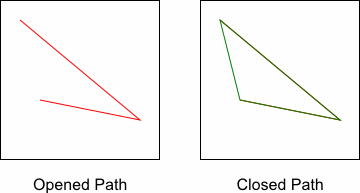### Converting to GraphicsPath

There can be times when you need to draw a Path instance on standard System.Drawing.Graphics. You can easily do that using the Path.ToGdiPlusGraphicsPath() method:

C#
```using (var bitmap = new Bitmap(160, 160, PixelFormat.Format24bppRgb, RgbColor.White))
using (var path = new Path())
{
path.MoveTo(20, 20);
path.LineTo(140, 120);
using (var graphics = bitmap.GetGdiPlusGraphics())
{
graphics.DrawPath(new System.Drawing.Pen(RgbColor.Black), path.ToGdiPlusGraphicsPath());
}
}
```

### Serializing Path

The Path class is marked with the Serializable attribute. This means that you can save an instance of the Path class to a file and then restore it for further editing. The following snippet demonstrates how to serialize the path:

C#
```using (var path = new Path())
{
path.MoveTo(10, 60);
path.CurveTo(new System.Drawing.PointF(40, 20), new System.Drawing.PointF(70, 60));

IFormatter formatter = new System.Runtime.Serialization.Formatters.Binary.BinaryFormatter();
System.IO.Stream stream = new System.IO.FileStream("MyFile.path",
System.IO.FileMode.Create, System.IO.FileAccess.Write, System.IO.FileShare.None);
formatter.Serialize(stream, path);
stream.Close();
}
```

To restore the path back to its former state use the following code:

C#
```IFormatter formatter = new System.Runtime.Serialization.Formatters.Binary.BinaryFormatter();
System.IO.Stream stream = new System.IO.FileStream("MyFile.path",